##Homographic Relation Example

The basics for homographic relations between two real variables are discussed in HomographicRelation.html . Here is the construction of such a relation based on a conic (the ellipse below) and two fixed tangents (a, b) of it. The relation is defined between the line coordinate x of a and the line coordinate y of b, using the following recipe:
For an arbitrary point C of the conic, consider the tangent (c) at C and its intersection points X(x) with (a) and Y(y) with (b). The correspondence y = f(x) between the line coordinates is a homographic relation. The figure below displays the graph of this function, which is a rectangular hyperbola, together with its asymptotic lines.
Note the location of the asymptotics corresponding to points B', C' symmetric to B, C w.r. to the center of the conic.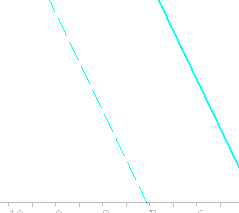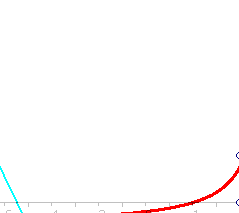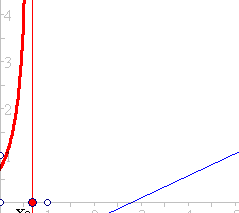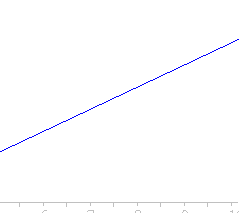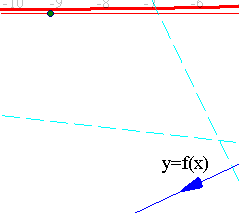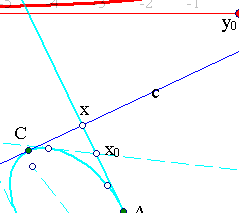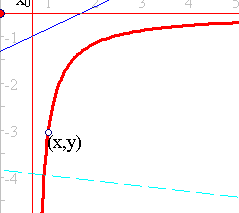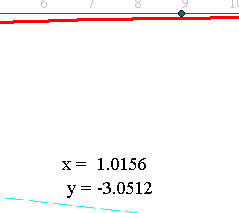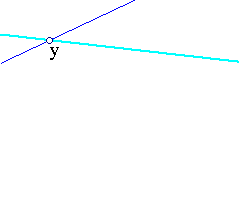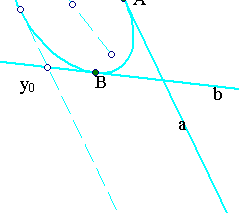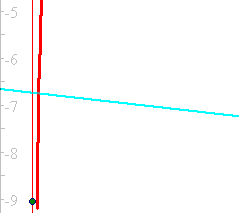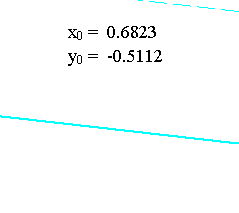Note that this is, in some sense, the inverse of the construction of the conic as an envelope of lines, discussed in the file Chasles_Steiner_Envelope.html .